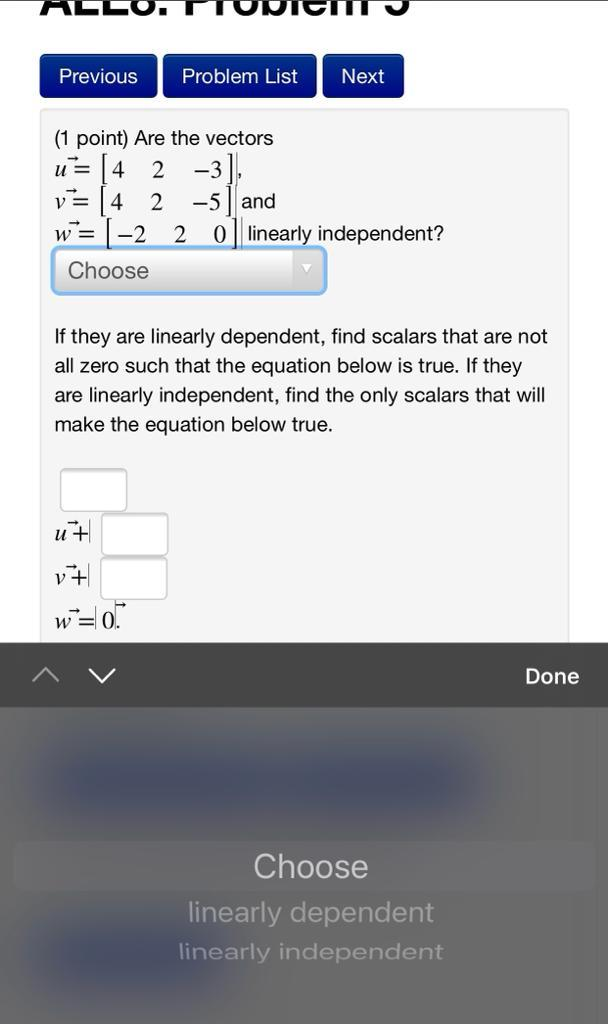### Create an Account

Already have account?

### Forgot Your Password ?

Home / Questions / Previous Problem List Next (1 point) Are the vectors U= [4 2 -3] V= 4. 2 -5 and W= -2 2 o ...

# Previous Problem List Next (1 point) Are the vectors U= [4 2 -3] V= 4. 2 -5 and W= -2 2 o linearly independent? Choose If they are linearly dependent, find scalars that are not all zero such that the

Previous Problem List Next (1 point) Are the vectors U= [4 2 -3] V= 4. 2 -5 and W= -2 2 o linearly independent? Choose If they are linearly dependent, find scalars that are not all zero such that the equation below is true. If they are linearly independent, find the only scalars that will make the equation below true. u7 17 w = 0 Done Choose linearly dependent linearly independentApr 15 2021 View more View Less

#### Answer (Solved)Subscribe To Get Solution